# IBPS Clerk Mains 2017 – Quantitative Aptitude DAY 8

Dear Banking Aspirants,

IBPS CLERK Mains 2017, the last exam of the year 2017 is going to held in 21.01.2018.There is only few days in our hands for preparation and you have to work hard to achieve your goal, because the competition is very high as per the vacancy is concerned.

So start your preparation now with this study Planner for IBPS Clerk Mains. It’s a 20 days complete planner with various Aptitude, Reasoning, English, Current affairs & Static Gk topics Included. There are 4 Mock tests available in this planner.

We have planned this Planner in Such a way that this will cover the entire current affairs quiz from the month of October to January (1st week). Because Banking Awareness, Current Affairs, Static Gk & Computer Awareness will play a vital role in cracking the IBPS Clerk Mains exam. Though the exam is getting tougher and tougher for each and every Year, Only consistently hard working candidates will crack the exam. So be a continuous hard worker and grab the opportunity to achieve your success.

### Make hay while the Sunshine!!!!!!!!!!!

So as per the planner here is content related to Quantitative Aptitude .

IBPS CLERK Mains 2017 -Quantitative Aptitude DAY 8

Quantitative Aptitude

Time: 20 Minutes

D.1-5) In the following two equation are given I and II. By solving those equations give corresponding answer.

Q.1)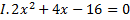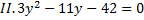a)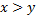b)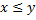c)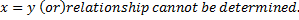d)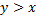e)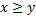Q.2)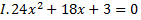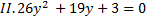a)b)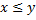c)d)e)Q.3)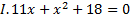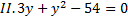a)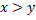b)c)d)e)Q.4)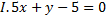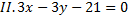a)b)c)d)e)Q.5)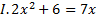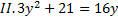a)

b)c)d)e)D.6-10) In each of these questions, two equations (I) and (II) are given. You have to solve both the equations and give answer.

Q.6)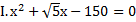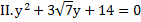a) x > y

b) x < y

c) x ≥ y

d) x ≤ y

e) x = y or relation cannot be established between ‘x’ and ‘y’.

Q.7) I.3x2-x-10=0

II.3x2-x-2=0

a) x > y

b) x < y

c) x ≥ y

d) x ≤ y

e) x = y or relation cannot be established between ‘x’ and ‘y’.

Q.8) I.x2+13x+40=0

II.y2+7y+12=0

a) x > y

b) x < y

c) x ≥ y

d) x ≤ y

e) x = y or relation cannot be established between ‘x’ and ‘y’.

Q.9) I. x2-30x +216=0

y2-29y+210=0

a) x > y

b) x < y

c) x ≥ y

d) x ≤ y

e) x = y or relation cannot be established between ‘x’ and ‘y’.

Q.10) I. x2-30x +221=0

y2-36y+323=0

a) x > y

b) x < y

c) x ≥ y

d) x ≤ y

e) x = y or relation cannot be established between ‘x’ and ‘y’.

Q.11)A started a business with an amount of Rs.20000, after 6 months B joined  him by investing  Rs.5000 and after another 3 months B invests Rs.5000 and C invests Rs.15000 for 3 months. If they received a total profit of Rs.15400.Find the  sum of A and B share

a) 20100

b) 13300

c) 10100

d) 20300

e) None of these

Q.12) A, B and C enter into a business with investment of Rs.8000, Rs.7500 and Rs.9000 respectively. After 1 year A invests Rs.2000 and C withdraws Rs.1500, find the profit ratio at the end of 2 years?

a) 13:14:17

b) 11:13:10

c) 13:9:11

d) 12:10:11

e) 15:12:13

Q.13) Three persons A, B and C start a business with the investment ratio of 4:3:6. After 3 months A withdraws half of his initial investment and after 9 months C withdraws half of his initial investment. If the total profit at the end of one year is Rs.21500.Find the share of A?

a) Rs.4800

b) Rs.6000

c) Rs.5000

d) Rs.5500

e) Rs.4500

Q.14) Three friends A, B, and C started a venture with capitals in the ratio 6:2:7. At the end every 4 months A halves his capital available at the material time, whereas B doubles his capital available at the material time and C leaves his capital untouched. This process is repeated till the end of the year. If at the end of the year B’s share of the profits is Rs.10000, what is the total profit?

a) Rs.30500

b) Rs.42500

c) Rs.32500

d) Rs.40500

e) Rs.31500

Q.15) A and B entered into the business and invested Rs.600 and Rs.500 respectively. After four months, person A replaced by person C, and he invested x% of A invested. At the end of the year, total profit is Rs.2160 and C’s profit share is Rs.480. What is the value of X?

a) 40

b) 45

c) 50

d) 48

e) None of these

Q.1) c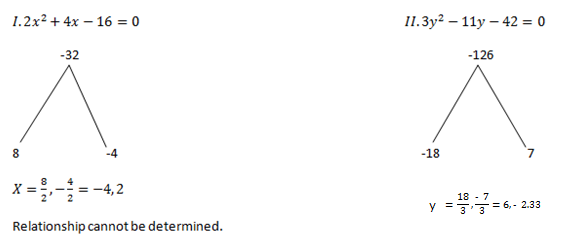Q.2) e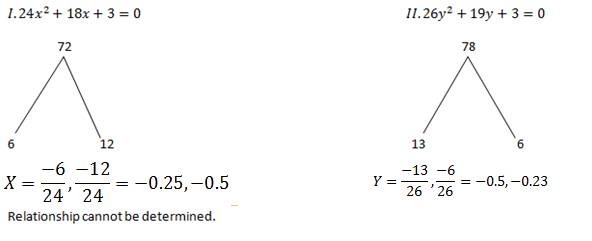Q.3) b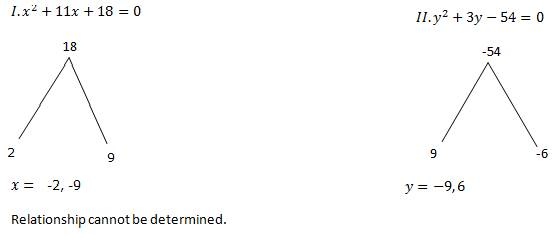Q.4) a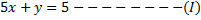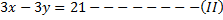Solve the above equation and get the X and Y values,

X=2

Y=-5

Q.5) c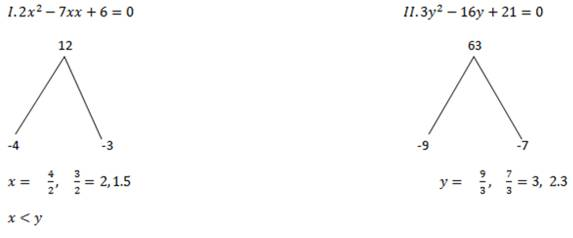Q.6) e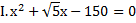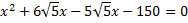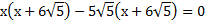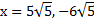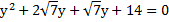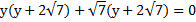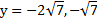Hence relationship cannot be determined.

Q.7) e

I.3x2-x-10=0

-30

-6/3        5/3

x= 2, -1.666

II.3x2-x-2=0

-6

-3/3       2/3

y= 1, -0.666

Relationship cannot be determined.

Q.8) b

I.x2+13 x+40=0

40

8             5

X=-8, -5

II.y2+7y+12=0

12

3             4

y=-3, -4

y>x

Q.9) e

x2-30x +216=0

216

-12        -18

x=12, 18

y2-29y+210=0

210

-14         -15

y=14, 15

Relationship cannot be determined

Q.10) d

x2-30x +221=0

221

-13        -17

x=13, 17

y2-36y+323=0

323

-17        -19

y=17, 19

x ≤ y

Q.11) b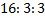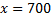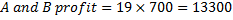Q.12) d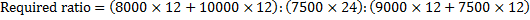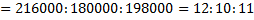Q.13) c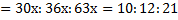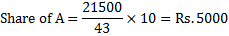Q.14) Ratio of their investments for 1 year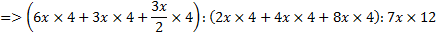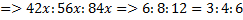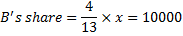Total profit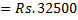Q.15)c

A : B : C =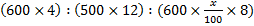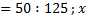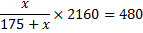X = 50

You can join in our Telegram Channel the invite given below and you can share this with your friends and other aspirants who are preparing for their exams like you.

Caution: You should have Telegram app installed in your mobile or laptop to join the group. If you don’t have telegram app in your mobile. First install the app and click the link given below….